# Search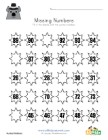## Groundhog Day Missing Numbers Worksheet

Fill in the missing numbers in each of the suns.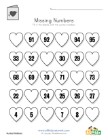## Valentine's Day Missing Numbers Worksheet

Fill in the missing numbers in each of the hearts.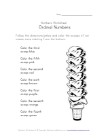## Practice Ordinal Numbers

Follow the directions and color each of the sco...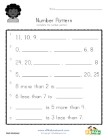## Number Pattern Worksheet 3

Fill in the blanks to complete the number patte...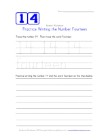## Practice Writing the Number Fourteen

Practice writing the number 14 and the word fou...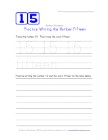## Practice Writing the Number Fifteen

Practice writing the number 15 and the word fif...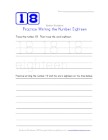## Practice Writing the Number Eighteen

Practice writing the number 18 and the word eig...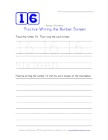## Practice Writing the Number Sixteen

Practice writing the number 16 and the word six...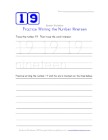## Practice Writing the Number Nineteen

Practice writing the number 19 and the word nin...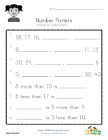## Number Pattern Worksheet 4

Fill in the blanks to complete the number patte...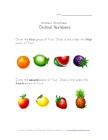## Identify the Ordinal Numbers

Kids are asked to circle and underline the appr...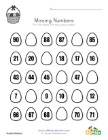## Easter Missing Numbers Worksheet

Fill in the missing numbers in each of the eggs.